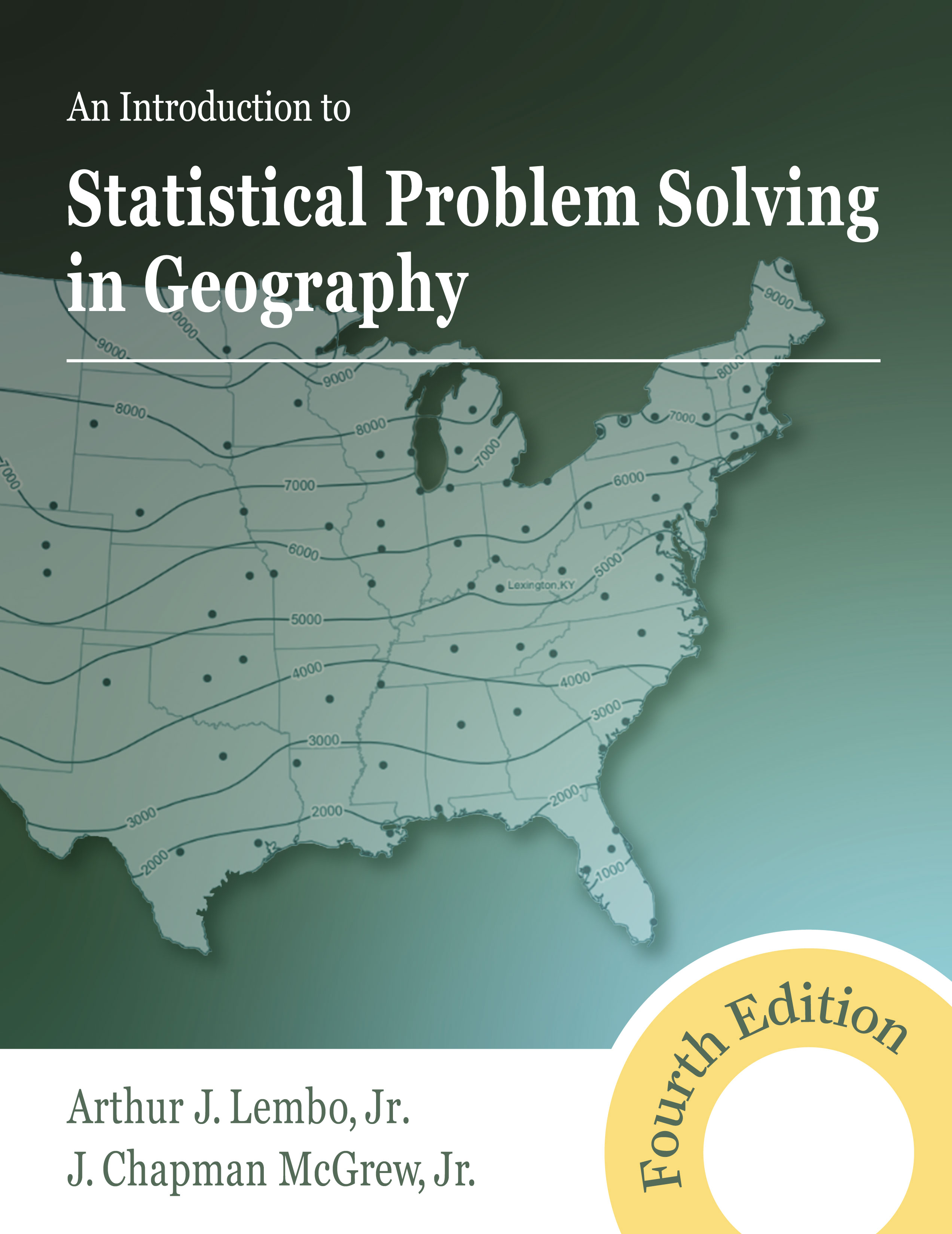354 pages, \$59.95 list
1-4786-4946-1
978-1-4786-4946-5
Instructor's resource materials forthcoming
eBook availability
An Introduction to Statistical Problem Solving in Geography
Fourth Edition
The fourth edition of An Introduction to Statistical Problem Solving in Geography continues its standing as the definitive introduction to statistics and quantitative analysis in geography. Assuming no reader background in statistics, the authors lay out the proper role of statistical analysis and methods in human and physical geography. They delve into the calculation of descriptive summaries and graphics to explain geographic patterns and use inferential statistics (parametric and nonparametric) to test for differences (t-tests, ANOVA), relationships (regression and correlation), and spatial statistics (point and area patterns, spatial autocorrelation).

This edition introduces more advanced topics, including logistic regression, two-factor ANOVA, and spatial estimation (inverse distance weighting, Kriging). Many chapters also include thought-provoking discussions of statistical concepts as they relate to the COVID-19 pandemic. Maintaining an exploratory and investigative approach throughout, the authors provide readers with real-world geographic issues and more than 50 map examples. Concepts are explained clearly and narratively without oversimplification. Each chapter concludes with a list of major goals and objectives. An epilogue offers over 150 open-ended geographic situations, inviting students to apply their new statistical skills to solve problems currently affecting our world.
Reactions
“This text seems to be exactly what I need. It strikes the right balance between rigor and accessibility. It contains some of the best explanations I could find for my students on key topics in statistics. The interesting practical applications supporting the core material and the numerous exercises at the end of the book are invaluable. It is the best textbook I could find on this subject at this level.” — Cristian Suteanu, Saint Mary’s University

“Rather than dreading statistics, this book shows students the value of a multitude of techniques and how to select the best one for a particular problem. Equally important, the authors show students how to interpret the results of their analysis.” — The Pennsylvania Geographer

“Social science statistics oftentimes is not focused on geography as a unique discipline with its own set of problems and applications. This text provides both the theory and application of statistics for undergraduate students in geography.” — Christopher Atkinson, University of North Dakota

“The book is great! A welcome resource for students who are just beginning geostatistics. The examples are contextually simple to understand without sacrificing too much theory.” — Peter Oduor, North Dakota State University

“This easy-to-understand book is a great resource for students. It is full of practical examples in plain English. The authors have presented and explained otherwise complicated topics of statistics in a manner that any college student can easily comprehend.” — Bhuiyan Alam, University of Toledo

"Without the McGrew text my course wouldn't be a 'geographic statistics' course. I love the book and my students do too." — Paul Sutton, University of Denver
Part 1: BASIC STATISTICAL CONCEPTS IN GEOGRAPHY
1. Introduction: The Context of Statistical Techniques
2. Geographic Data: Characteristics and Preparation

Part 2: DESCRIPTIVE PROBLEM SOLVING IN GEOGRAPHY
3. Descriptive Statistics and Graphics
4. Descriptive Spatial Statistics

Part 3: THE TRANSITION TO INFERENTIAL PROBLEM SOLVING
5. Basics of Probability and Discrete Probability Distributions
6. Continuous Probability Distributions and Probability Mapping
7. Basic Elements of Sampling
8. Estimation in Sampling

Part 4: INFERENTIAL PROBLEM SOLVING IN GEOGRAPHY
9. Elements of Inferential Statistics
10. Two-Sample and Dependent-Sample (Matched-Pairs) Difference Tests
11. Three-or-More-Sample Difference Tests: Analysis of Variance Methods
12. Categorical Difference Tests

Part 5: INFERENTIAL SPATIAL STATISTICS
13. General Issues in Inferential Spatial Statistics
14. Point Pattern Analysis
15. Area Pattern Analysis

Part 6: STATISTICAL RELATIONSHIPS BETWEEN VARIABLES
16. Correlation
17. Simple Linear Regression

Part 7: MULTIVARIATE PROBLEM-SOLVING IN GEOGRAPHY
18. Examples of Multivariate Problem-Solving in Geography
19. Multifactor Analysis: Two-Way Analysis of Variance
20. The Basics of Binary Logistic Regression
21. Estimation with Spatial Data

Part 8: EPILOGUE
22. Problem Solving and Policy Determination in Practical Geographic Situations

Appendix: Statistical Tables